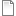# Age-class model of population dynamics, using multiple-instance submodel

ModelId:
ageclass5
SimileVersion:
5.9

This models a population of animals in terms of four age-classes. For each age-class, we use a compartment (state variable) to represent the number of individuals in that class.

This version uses a four-instance submodel to represent the four age-classes. The ageing flow (from one class to the next) thus has to be handled by transferring the value for the outflow from each class to be assigned to the inflow for the next class. In this model, this is done by placing all four outflow values in an array outside the submodel, then selecting the appropriate value from this array for each inflow.Equations:

Equations in Desktop

births = sum([these_births])
pop size = sum([pop_size])
ageing = [ageing_out]

Equations in Age class
pop size: initial value = element([2,0,0,0],index(1))
births = if index(1) == 1 then births else 0
ageing out = element([1,0.2,0.1,0],index(1))*pop_size
deaths = m*pop_size
ageing in = if index(1)>1 then element([ageing],index(1)-1)else 0
m = element([0.05,0.01,0.01,0.05],index(1))
r = element([0,0.05,0.2,0.1],index(1))*(1-pop_size/100)
these births= r*pop_size

Results:AttachmentSizeageclass5.sml53.95 KBageclass5.shf3.46 KB
Model tags: